Published: 15 May 2016

# Theoretical and bifurcation analysis of a flexible rotor supported by gas-lubricated bearing system with porous bushing

Cheng-Chi Wang1
Jui-Pin Hung2
1, 2Graduate Institute of Precision Manufacturing, National Chin-Yi University of Technology, Taichung, Taiwan
Corresponding Author:
Cheng-Chi Wang
Views 49

#### Abstract

The bifurcation behaviors of a flexible rotor supported by gas-lubricated bearing system with porous bushing are analyzed by a novel numerical method combining the finite difference method and differential transformation method. The results obtained by proposed method are verified with traditional finite difference method, and the analytical results by these two methods are consistent and also in good agreement. Furthermore, the dynamic orbits, power spectra, bifurcation diagram, Poincaré maps and maximum Lyapunov exponent are used to confirm the changes of rotor behavior as the rotor mass is increased. The results show that the rotor center reveals complex dynamic behaviors including periodic, sub-harmonic and chaotic motions. Especially, the rotor bearing system behaves chaos over the ranges of rotor mass 10.7 $\le {m}_{s}<$ 13.8 kg. The current results provide an effective means of the gas bearing systems and further understanding of the nonlinear dynamic behavior of bearing systems characterized by different rotor masses.

## 1. Introduction

Gas-lubricated bearing system with porous bushing (GBSP) is different from other air bearing system due its particularly attractive features such as low-noise rotation, high load capacity, improved damping properties and zero friction. GBSP is simpler in construction and cheaper than the externally pressurized bearings. The comparison with oil bearings and GBSP reveals that GBSP generates less heat and provides higher accuracy. GBSP are also applied in high speed spindles, and machine tools.

In 1964, Sneck and Yen  considered one-dimensional flow for radial direction in a porous medium and developed a perturbation solution for a finite journal bearing. Then, they verified theoretical solutions experimentally in 1965 .

In 2001, Sinha et al.  analyzed externally pressurized conical bearings with the porous constant gap when the slider rotated with uniform angular velocity. The system governing equations including coupled momentum and energy equations are analyzed by using finite difference method and various bearing characteristics are also determined. The results show that the inlet pressure decreases remarkably for highly porous surfaces, resulting in reduced load capacity of the bearing. But, the torque of system with respect to variation of permeability remains unaffected.

For the rotor dynamic study, the nonlinear dynamic behavior of rigid rotor supported by noncircular air journal bearing system including two, three and four-lobe bearings are solved by Rashidi et al. [4, 5] The Reynolds’ equation for air pressure distribution is analyzed by using finite element method and the rotor dynamic equations are calculated by Runge-Kutta method. The numerical results show that bifurcation phenomenon with different bearing numbers and rotor masses including periodic and non-periodic motions at different operational situations. It’s found that bearing number and rotor mass are the major parameters for the bearing system.

In 2010 to 2013 [6-9], Wang et al. studied various kinds of gas bearing and analyzed the bifurcation and dynamics behaviors avoiding the occurrence of fatigue or unexpected situations. For example, as united gas-lubricated bearings, the rotor center behaves a complex dynamic behavior under different values of the rotor mass and bearing number. The maximum Lyapunov exponent is also applied to distinguish different motions comprising periodic, sub-harmonic, quasi-periodic and chaotic responses. Furthermore, the dynamic behavior of noncircular air bearing system is studied by Wang et al. and a hybrid method including differential transformation method and finite difference method are proposed and used to solve the governing equations with various gas viscosity and rotational speed.

The remainder of this study is organized as follows. Section 2 develops a mathematical model of GBSP. Section 3 determines the Reynolds’ equation to obtain the gas pressure distribution by a hybrid method combining the finite difference method (FDM) and the differential transformation method (DTM). The numerical solutions are then compared with those obtained by traditional FDM. Section 4 presents the simulation results obtained using the proposed hybrid method for the vibrations of the rotor center for various rotor masses.

## 2. Governing equations

The GBSP system is used to form a closed cavity around the porous bushing with an appropriate casing and the porous material acts as a restrictor. The compressed gas is supplied and fed at a constant pressure, ${\stackrel{^}{P}}_{f}$, and the gas flows through the porous bushing into the bearing clearance. As the supply pressure drops to $\stackrel{^}{P}$, it exhausts into the atmosphere at pressure ${P}_{a}$. The GBSP model incorporates the ideal assumptions including the gas flow is assumed isothermal, the side flow of gas of the bearing is neglected, and the gas viscosity is constant. It is considered that a GBSP system comprising a perfectly-balanced flexible rotor supported symmetrically on two identical GBSPs mounting in turn on rigid pedestals. Due to the rotor is assumed as balancing perfectly and the GBSP is symmetric about its central axes, the system is confined to a single bearing supporting a rotor with two degrees of translatory oscillation in the transverse plane.

The gas pressure distribution between the rotor and the bushing is modeled by the non-dimensionalized Reynolds’ equation as follows:

1
$2\varsigma \mathrm{\Lambda }\beta \left(\frac{\partial {\stackrel{^}{P}}_{f}}{\partial \tau }-\frac{\partial {\stackrel{^}{P}}_{f}}{\partial \theta }\frac{\partial \varphi }{\partial \tau }\right)=\frac{{\partial }^{2}{\stackrel{^}{P}}_{f}}{\partial {\stackrel{^}{z}}^{2}},$
2
${\mathrm{\Lambda }}_{\varphi }{\frac{{\partial }^{2}{{\stackrel{^}{P}}_{f}}^{2}}{\partial {\stackrel{^}{z}}^{2}}|}_{\stackrel{^}{z}=1}+2\mathrm{\Lambda }\left(1-2\varsigma \frac{\partial \varphi }{\partial \tau }\right)\frac{\partial \left(\stackrel{^}{P}\stackrel{^}{h}\right)}{\partial \theta }+4\varsigma \mathrm{\Lambda }\frac{\partial \left(\stackrel{^}{P}\stackrel{^}{h}\right)}{\partial \tau }$
$=\frac{\partial }{\partial \theta }\left({\stackrel{^}{h}}^{3}\frac{\partial {\stackrel{^}{P}}^{2}}{\partial \theta }\right)+{\left(\frac{D}{L}\right)}^{2}{\stackrel{^}{h}}^{3}\frac{{\partial }^{2}{\stackrel{^}{P}}^{2}}{\partial {\stackrel{^}{z}}^{2}},$

where $\stackrel{^}{P}$ is the non-dimensionalized pressure, ${\stackrel{^}{P}}_{f}$ is the non-dimensionalized pressure in porous media, $\stackrel{^}{h}$ is the non-dimensionalized thickness of gas film, $\mathrm{\Lambda }$ is the bearing number and $\theta$, $\stackrel{^}{z}$ are the non-dimensionalized coordinates.

## 3. Simulation analysis by a novel numerical method

The simulation analysis is completed by two different numerical methods including the proposed method in this paper and traditional FDM. Accordingly, the FDM is applied for Eqs. (1) and (2) to discretize the $\theta$ and $\stackrel{^}{z}$ directions by the central-difference scheme and then the time domain $\tau$ by the implicit-back-difference scheme. Also, a uniform mesh size is used. In order to compare the calculation results and increase the accuracy of system, a novel numerical method is proposed in this study. It is commenced by applying the DTM to discretize the Reynolds’ equation given in Eqs. (1) and (2) with respect to time and thus Eqs. (1) and (2) become as Eqs. (3)-(6). Then, The FDM is used to discretize Eqs. (3)-(6) with respect to the $\theta$ and $\stackrel{^}{z}$ directions. Note that Eqs. (3)-(6) are discretized using the second-order-accurate central-difference scheme for both the first and the second derivatives. Substituting Eqs. (5)-(6) into Eqs. (3)-(4) yields Eqs. (7), (8):

3
$2\varsigma \mathrm{\Lambda }\beta \frac{\partial {\stackrel{^}{P}}_{f}}{\partial \tau }-2\varsigma \mathrm{\Lambda }\beta \frac{\partial \varphi }{\partial \tau }\otimes \frac{\partial {\stackrel{^}{P}}_{f}}{\partial \theta }=\frac{{\partial }^{2}{\stackrel{^}{P}}_{f}}{\partial {\stackrel{^}{z}}^{2}},$
4
${\mathrm{\Lambda }}_{\varphi }{\frac{{\partial }^{2}{{\stackrel{^}{P}}_{f}}^{2}}{\partial {\stackrel{^}{z}}^{2}}|}_{\stackrel{^}{z}=1}+2\mathrm{\Lambda }\frac{\partial \stackrel{^}{P}}{\partial \theta }\otimes \stackrel{^}{h}+2\mathrm{\Lambda }\frac{\partial \stackrel{^}{h}}{\partial \theta }\otimes \stackrel{^}{P}-4\mathrm{\Lambda }\varsigma \frac{\partial \varphi }{\partial \tau }\otimes \frac{\partial \stackrel{^}{P}}{\partial \theta }\otimes \stackrel{^}{h}$
$\begin{array}{c}-4\varsigma \mathrm{\Lambda }\frac{\partial \varphi }{\partial \tau }\otimes \frac{\partial \stackrel{^}{h}}{\partial \theta }\otimes \stackrel{^}{P}+4\mathrm{\Lambda }\varsigma \frac{\partial \stackrel{^}{P}}{\partial \tau }\otimes \stackrel{^}{h}+4\varsigma \mathrm{\Lambda }\frac{\partial \stackrel{^}{h}}{\partial \tau }\otimes \stackrel{^}{P}\end{array}$
$=\frac{\partial J}{\partial \theta }\otimes \frac{\partial Q}{\partial \theta }+J\otimes \frac{{\partial }^{2}Q}{{\partial }^{2}\theta }+{\left(\frac{D}{L}\right)}^{2}J\otimes \frac{{\partial }^{2}Q}{\partial {\stackrel{^}{z}}^{2}},$

where:

5
$J\left(k\right)={\stackrel{^}{h}}^{3}=\stackrel{^}{h}\otimes \stackrel{^}{h}\otimes \stackrel{^}{h}={\sum }_{l=0}^{k}{\stackrel{^}{h}}_{i,j}\left(k-l\right){\sum }_{m=0}^{l}{\stackrel{^}{h}}_{i,j}\left(l-m\right){\stackrel{^}{h}}_{i,j}\left(m\right),$
6
$Q\left(k\right)={\stackrel{^}{P}}^{2}=\stackrel{^}{P}\otimes \stackrel{^}{P}={\sum }_{l=0}^{k}{\stackrel{^}{P}}_{i,j}\left(k-l\right){\stackrel{^}{P}}_{i,j}\left(l\right).$

The governing equations become:

7
$2\varsigma \mathrm{\Lambda }\beta \left(\frac{k+1}{\stackrel{~}{H}}\right){\left({\stackrel{^}{P}}_{f}\right)}_{i,j}\left(k+1\right)$
$\mathrm{}\mathrm{}\mathrm{}\mathrm{}\mathrm{}\mathrm{}-2\varsigma \mathrm{\Lambda }\beta {\sum }_{l=0}^{k}\left(\frac{k+1}{\stackrel{~}{H}}\right)\left(\frac{{\left({\stackrel{^}{P}}_{f}\right)}_{i+1,j}\left(k-l\right)-{\left({\stackrel{^}{P}}_{f}\right)}_{i-1,j}\left(k-l\right)}{2\mathrm{\Delta }\theta }\right){\varphi }_{i,j}\left(l+1\right)$
$\mathrm{}\mathrm{}\mathrm{}\mathrm{}\mathrm{}\mathrm{}=\left(\frac{{\left({\stackrel{^}{P}}_{f}\right)}_{i,j+1}\left(m\right)-2{\left({\stackrel{^}{P}}_{f}\right)}_{i,j}\left(m\right)+{\left({\stackrel{^}{P}}_{f}\right)}_{i,j-1}\left(m\right)}{{\left(\mathrm{\Delta }\stackrel{^}{z}\right)}^{2}}\right),$
8
${\mathrm{\Lambda }}_{\varphi }{\left(\frac{{\left({{\stackrel{^}{P}}_{f}}^{2}\right)}_{i,j+1}\left(m\right)-2{\left({{\stackrel{^}{P}}_{f}}^{2}\right)}_{i,j}\left(m\right)+{\left({{\stackrel{^}{P}}_{f}}^{2}\right)}_{i,j-1}\left(m\right)}{{\left(\mathrm{\Delta }\stackrel{^}{z}\right)}^{2}}\right)|}_{\stackrel{^}{z}=1}$
$\mathrm{}\mathrm{}\mathrm{}\mathrm{}\mathrm{}\mathrm{}+2\mathrm{\Lambda }{\sum }_{l=0}^{k}{\stackrel{^}{h}}_{i,j}\left(k-l\right)\left(\frac{{\stackrel{^}{P}}_{i+1,j}\left(l\right)-{\stackrel{^}{P}}_{i-1,j}\left(l\right)}{2\mathrm{\Delta }\theta }\right)+2\mathrm{\Lambda }{\sum }_{l=0}^{k}{\stackrel{^}{P}}_{i,j}\left(k-l\right)\left(\frac{{\stackrel{^}{h}}_{i+1,j}\left(l\right)-{\stackrel{^}{h}}_{i-1,j}\left(l\right)}{2\mathrm{\Delta }\theta }\right)$
$\mathrm{}\mathrm{}\mathrm{}\mathrm{}\mathrm{}\mathrm{}-4\mathrm{\Lambda }\varsigma {\sum }_{l=0}^{k}{\stackrel{^}{h}}_{i,j}\left(k-l\right){\sum }_{m=0}^{l}\left[\left(\frac{k+1}{\stackrel{~}{H}}\right)\left(\frac{{\stackrel{^}{P}}_{i+1,j}\left(m\right)-{\stackrel{^}{P}}_{i-1,j}\left(m\right)}{2\mathrm{\Delta }\theta }\right){\varphi }_{i,j}\left(l-m\right)\right]$
$\mathrm{}\mathrm{}\mathrm{}\mathrm{}\mathrm{}\mathrm{}-4\mathrm{\Lambda }\varsigma {\sum }_{l=0}^{k}{\stackrel{^}{P}}_{i,j}\left(k-l\right){\sum }_{m=0}^{l}\left[\left(\frac{k+1}{\stackrel{~}{H}}\right)\left(\frac{{\stackrel{^}{h}}_{i+1,j}\left(m\right)-{\stackrel{^}{h}}_{i-1,j}\left(m\right)}{2\mathrm{\Delta }\theta }\right){\varphi }_{i,j}\left(l-m\right)\right]$
$\mathrm{}\mathrm{}\mathrm{}\mathrm{}\mathrm{}\mathrm{}-4\mathrm{\Lambda }\varsigma {\sum }_{l=0}^{k}\left[\left(\frac{k+1}{\stackrel{~}{H}}\right){\stackrel{^}{h}}_{i,j}\left(k-l\right){\stackrel{^}{P}}_{i,j}\left(l+1\right)\right]+4\mathrm{\Lambda }\varsigma {\sum }_{l=0}^{k}\left[\left(\frac{k+1}{\stackrel{~}{H}}\right){\stackrel{^}{P}}_{i,j}\left(k-l\right){\stackrel{^}{h}}_{i,j}\left(l+1\right)\right]$
$\mathrm{}\mathrm{}\mathrm{}\mathrm{}\mathrm{}\mathrm{}={\sum }_{l=0}^{k}\left(\frac{{J}_{i+1,j}\left(k-l\right)-{J}_{i-1,j}\left(k-l\right)}{2\mathrm{\Delta }\theta }\right)\left(\frac{{Q}_{i+1,j}\left(l\right)-{Q}_{i-1,j}\left(l\right)}{2\mathrm{\Delta }\theta }\right)$
$\mathrm{}\mathrm{}\mathrm{}\mathrm{}\mathrm{}\mathrm{}+{\sum }_{l=0}^{k}{J}_{i,j}\left(k-l\right)\left(\frac{{Q}_{i,j+1}\left(l\right)-2{Q}_{i,j}\left(l\right)+{Q}_{i,j-1}\left(l\right)}{{\left(\mathrm{\Delta }\mathrm{I}\right)}^{2}}\right)$
$\mathrm{}\mathrm{}\mathrm{}\mathrm{}\mathrm{}\mathrm{}+{\left(\frac{D}{L}\right)}^{2}{\sum }_{l=0}^{k}{J}_{i,j}\left(k-l\right)\left(\frac{{Q}_{i,j+1}\left(l\right)-2{Q}_{i,j}\left(l\right)+{Q}_{i,j-1}\left(l\right)}{{\left(\mathrm{\Delta }\stackrel{^}{z}\right)}^{2}}\right).$

The calculation of iterative procedure can be integrated as follows. First, the new values of acceleration, velocity, and displacement of rotor are obtained by following a time increment $\mathrm{\Delta }\tau$. Second, from first step the rotor center displacements can then be determined and the corresponding change of the gas film gap ($\stackrel{^}{h}$) can be analyzed. Then the new value of $\stackrel{^}{h}$ is substituted into governing dequations to give the new pressure distribution of the gap between the shaft and the bushing. Third, the pressure distribution obtained from second step is integrated to calculate the internal force caused by the gas-lubricated film. Finally, the displacement and velocity values calculated in the first step, the gas pressure computed in the second step, and the internal force analyzed in the third step are taken as the new initial conditions. Applying this new set of conditions, the calculation procedure returns to the first step to calculate the changes in the GBSP system during the time interval $\mathrm{\Delta }\tau \to 2\mathrm{\Delta }\tau$. These data including the orbital paths and velocity of the rotor center are used to generate bifurcation diagrams and Poincaré maps.

## 4. Numerical results

Table 1 presents the Poincaré maps obtained by the FDM and DTM&FDM for the orbits of the rotor center. It is observed that a good agreement by DTM&FDM method exists between the two sets of results at different rotor mass values. It also compares with different values of the time step. It can be seen that the orbits are in agreement to approximately 4 decimal places for the different time steps especially obtained by DTM&FDM method as shown in Table 1 and 2.

Table 1Comparison of rotor center orbits calculated by FDM and DTM&FDM methods, respectively

 Displacement conditions X2(nT) Time step: 0.001 Time step: 0.01 FDM ${m}_{r}=$7.2 kg$\omega =$1980 rad/s 0.008823820432 0.008961092741 DTM&FDM 0.008825114313 0.008824802704 FDM ${m}_{r}=$10.1 kg$\omega =$1980 rad/s 0.059034858544 0.058062840042 DTM&FDM 0.059136319514 0.059136084996

Table 2Poincaré maps with different time increments and rotor mass by DTM&FDM

 ${m}_{r}=$ 7.2 kg ${m}_{r}=$ 10.1 kg $\tau$ X2(nT) Y2(nT) $\tau$ X2(nT) Y2(nT) $\pi$/300 0.008823943 –0.060185357 $\pi$/300 0.059136649 –0.075228092 $\pi$/600 0.008823325 –0.060185087 $\pi$/600 0.059136640 –0.075228387

Fig. 1Dynamic orbits of rotor center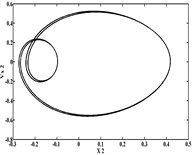a)${m}_{r}=$ 9.7 kg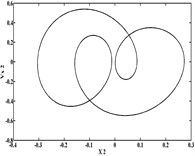b)${m}_{r}=\mathrm{}$9.96 kg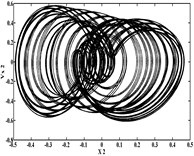c)${m}_{r}=\mathrm{}$10.8 kg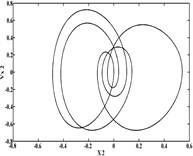d)${m}_{r}=\mathrm{}$13.5 kg

Figs. 1 and 2 show that the orbits and power spectra of the rotor center are regular at low values of the rotor mass (${m}_{r}=$9.7 and 9.96 kg), but become irregular at ${m}_{r}=$10.8 kg. This irregular behavior diverge as non-symmetric and non-periodic motion. When the rotor mass is increased at ${m}_{r}=$13.5 kg, the system converges to periodic motion.

Fig. 2Power spectra of rotor center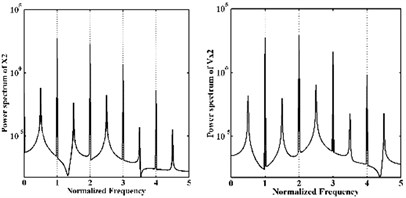a)${m}_{r}=$ 9.7 kg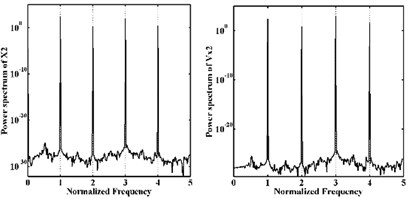b)${m}_{r}=$9.96 kg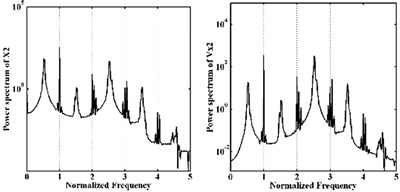d)${m}_{r}=$10.8 kg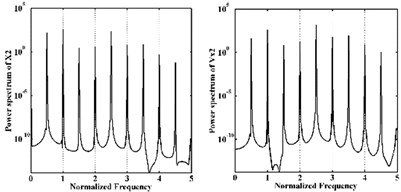e)${m}_{r}=$13.5 kg

Fig. 3Bifurcation diagrams versus rotor mass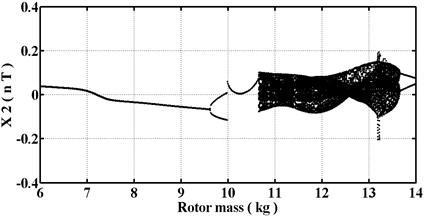a) X2(nT)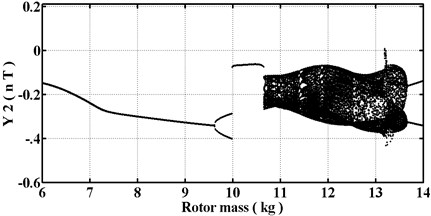b) Y2(nT)

Fig. 3 presents the bifurcation diagrams and shows the rotor center displacement of GBSP against the rotor mass. It is observed that qualitatively different behavior is varied at different values of ${m}_{r}$ within the range 6.0 to 14.0 kg. Fig. 4 present the Poincaré maps at ${m}_{r}=$ 9.7, 9.96, 10.8, and 13.5 kg, respectively. The dynamic motion of the rotor center is $T$-periodic at lower values of the rotor mass, i.e. ${m}_{r}<$9.7 kg. However, the periodic motion loses its stability at ${m}_{r}=$9.7 kg and is replaced by sub-harmonic motion of 2$T$ as shown in Fig. 3 and Fig. 4(a). This sub-harmonic is persisted over the interval 9.7$\le {m}_{r}<$9.96, but when the mass is increased to ${m}_{r}=$9.96 kg, the sub-harmonic motion is changed to a $T$-periodic motion in the $x$- and $y$-directions shown in Fig. 3 and Fig. 4(b).

When the mass is increased to ${m}_{r}=$10.8 kg, system is changed to chaotic motion and maintained over the interval 10.8$\le {m}_{r}<$13.5 kg. The chaotic situation is shown in Fig. 3 and proved in Fig. 4(c). However, the chaotic motion transferred its motion at a rotor mass of 13.5 kg and is bifurcated into a 2$T$-periodic motion shown in Fig. 3 and Fig. 4(d). Then, the sub-harmonic of 2$T$ motion is behaved over the interval 13.5$\le {m}_{r}\le$14.0 kg. It can be seen that two discrete points in the Poincaré maps at ${m}_{r}=$ 9.7 and 13.5 kg mean 2$T$ sub-harmonic motion and non-order discrete points at ${m}_{r}=$10.8 kg reveal chaos.

In order to further verify the occurrence of chaotic motion, the maximum Lyapunov exponent is applied and it is shown that a positive value (as ${m}_{r}$ equals 10.8) presents chaos over the intervals 10.8$\le {m}_{r}<$13.5 kg shown in Fig. 5(a) and equals zero which means system behaves periodic motion. From the discussions above, it is evident that the behavior of the rotor center is dependent on the rotor mass. Table 3 summarizes the motions performed by the rotor center for rotor mass values in the range 6.0$\le {m}_{r}\le$14.0 kg.

Fig. 4Poincaré maps of rotor center trajectories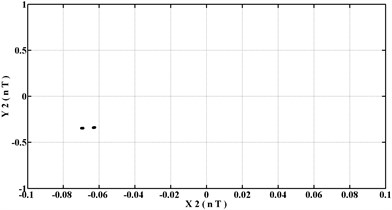a)${m}_{r}=$ 9.7 kg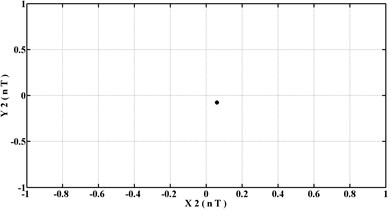b)${m}_{r}=$9.96 kg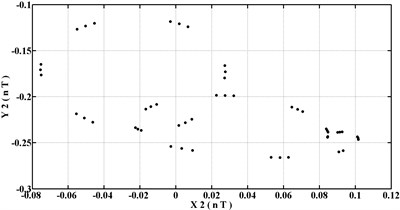c)${m}_{r}=$10.8 kg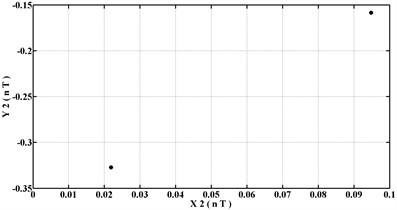d)${m}_{r}=$13.5 kg

Fig. 5Maximum Lyapunov exponents of system at different values of rotor mass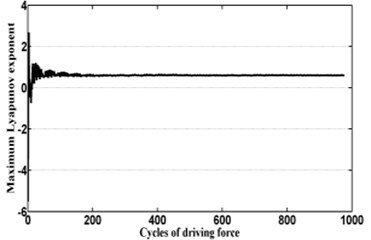a)${m}_{r}=$10.8 kg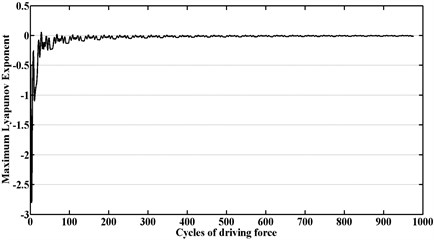b)${m}_{r}=$ 9.7 kg

Table 3Variation of rotor center response with rotor mass over interval 6.0 ≤mr≤ 14.0 kg

 Rotor mass [6.0, 9.7) [9.7, 9.96) [9.96, 10.8) [10.8, 13.5) [13.5, 14.0) Behavior $T$ 2$T$ $T$ Chaotic 2$T$

## 5. Conclusions

In this paper, the bifurcation behaviors of a flexible rotor supported by gas-lubricated bearing system with porous bushing (GBSP) are analyzed and the chaotic behavior has also been found by utilizing a hybrid numerical scheme comprising the differential transformation method and the finite difference method in this paper. The system orbits, power spectra, bifurcation diagrams, Poincaré maps and maximum Lyapunov exponent have revealed the presence of a complex dynamic behavior comprising periodic, sub-harmonic and chaotic responses of the rotor center. From Table 1 the results obtained by the FDM and proposed hybrid method for the orbits of the rotor center prove that a good agreement exists between different sets of results. For the results of nonlinear dynamic motion, this paper provide a fully understanding of GBSP systems characterized by different rotor masses. Specifically, the results have shown that system exists chaotic motion over the ranges of rotor mass 10.8$\le {m}_{r}<$13.5 kg under rotational speed of 1980 rad/s.

#### Cited by

Electrical Performance Safety Detection of Aramid Casing Based on Molecular Dynamics and Deep Learning Algorithm
Modeling and analysis of dynamic behaviors for a flexible rotor system supported in tilting pad aerodynamic bearings
Role of artificial intelligence in rotor fault diagnosis: a comprehensive review
APPLICATION OF MAGNETOELASTIC BEARINGS FOR DAMPING OF TURBOEXPANDER ROTOR VIBRATIONS
Nonlinear dynamics study of a high-temperature rotor-bearing-seal system in gas turbine

06 October 2015
Accepted
20 December 2015
Published
15 May 2016
SUBJECTS
Chaos, nonlinear dynamics and applications
Keywords
bifurcation
porous bushing
chaotic
Acknowledgements

The financial support of this research by Ministry of Science and Technology, under the Projects Nos. MOST 103-2622-E-167-015-CC3 and 103-2221-E-167-011 are greatly appreciated.